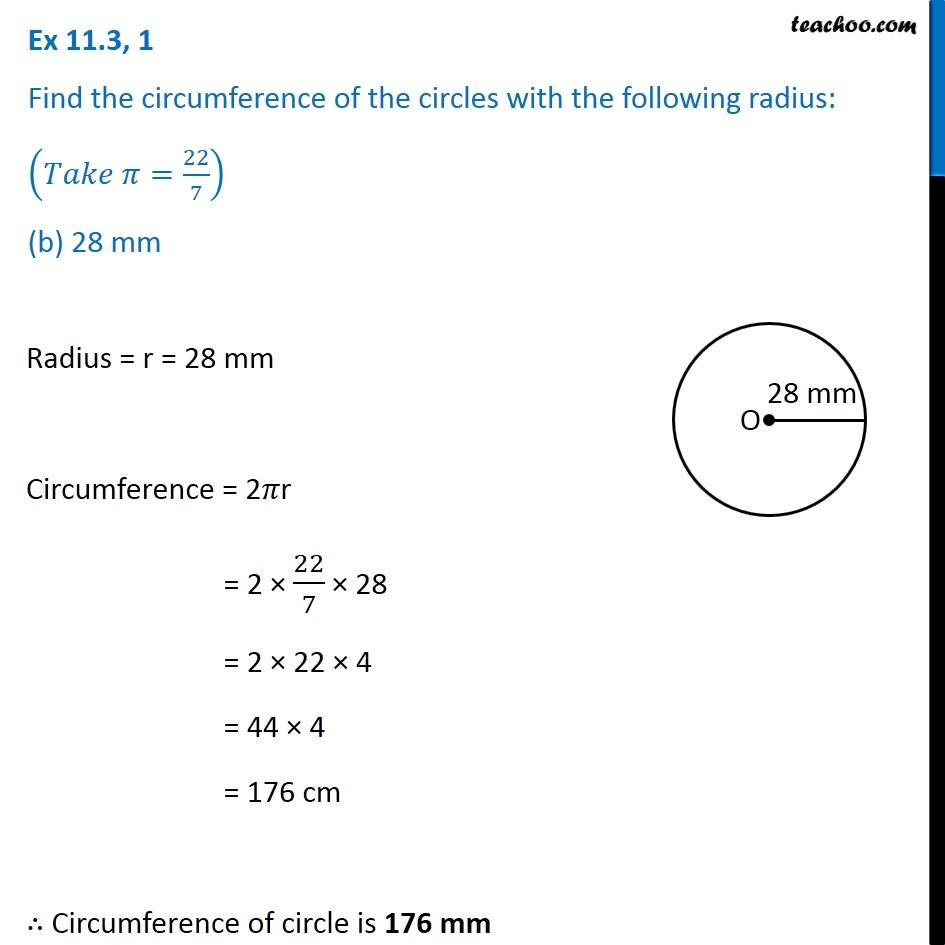1. Chapter 11 Class 7 Perimeter and Area
2. Serial order wise
3. Ex 11.3

Transcript

Ex 11.3, 1 Find the circumference of the circles with the following radius: (𝑇𝑎𝑘𝑒 𝜋=22/7) (b) 28 mm Radius = r = 28 mm Circumference = 2𝜋r = 2 × 22/7 × 28 = 2 × 22 × 4 = 44 × 4 = 176 cm ∴ Circumference of circle is 176 mm

Ex 11.3

Chapter 11 Class 7 Perimeter and Area
Serial order wise